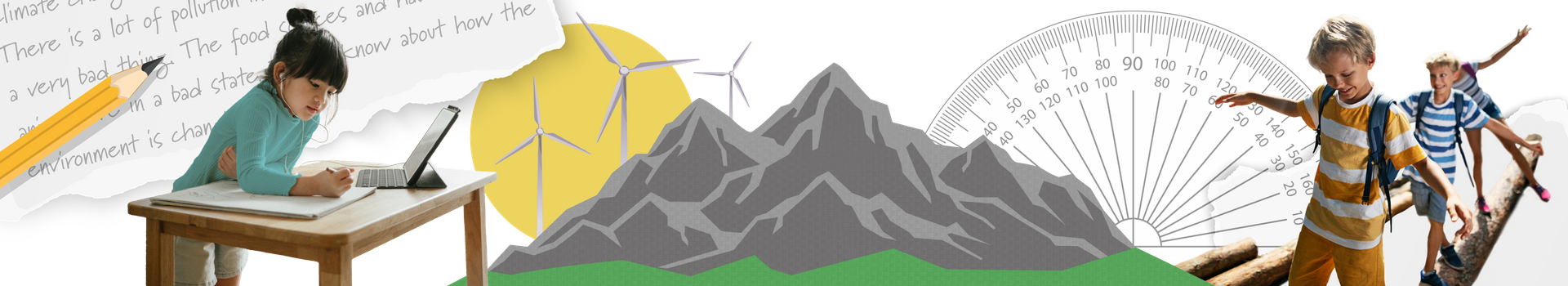In Grade 6 Mathematics, our students will round out their junior math journey by exploring very large numbers, operations, and measurement through sports and games, and discovering how fractions, decimal numbers, and percentages are applied in everyday settings. They will also connect algebra to science, learn about different payment methods in financial literacy, and continue exploring how code is controlled in different ways. This course is designed to be 200-300 instructional hours.

Curriculum Information: Mathematics (2020)

Developed by: D2L

Development Date: 2020

Please note that elementary courses do not have prerequisites, and Ontario schools do not issue transcript credits at the elementary level. All courses are available in the facilitated and independent pathways.

Facilitated

Independent

#### Course Outline

Numbers

In the Numbers unit, students read and write numbers up to 1 000 000, understand, represent, compare, and order numbers, and solve word problems up to 1 000 000. Students identify prime and composite numbers and find the prime factors for composite numbers. Students also understand place value and represent, compare, and order decimal numbers.

Geometry

In the Geometry unit, students sort and classify quadrilaterals, sort and construct polygons, and classify, measure, and construct angles. They learn how to find unknown angles. Students build 3D models using drawings and sketch isometric perspectives and different views. Furthermore, students plot and read points on a Cartesian plane.

Operations

In the Operations unit, students multiply and divide four-digit numbers by two-digit numbers, add and subtract decimal numbers to thousandths, and multiply and divide decimal numbers by whole numbers using concrete materials and algorithms.

Fractions, Percentages, Rations, and Unit Rates

In the Fractions, Percentages, Ratios, and Unit Rates unit, students represent fractions, compare fractions and mixed numbers using tools and fraction notation, and order fractions and mixed numbers. Students add and subtract fractions and multiply and divide whole numbers by proper fractions. They solve problems involving rations and learn how to calculate the percentage of a whole numbers. Students learn about the relationships among percentages, decimals, and fractions and explore the concept of unit rates.

Data Management and Probability

In the Data Management and Probability unit, students learn about discrete and continuous data, collect and organize data, select appropriate graphs, use technology, and determine how well a set of data represents a population. They learn how to create an infographic to represent data. Furthermore, students express theoretical probability as a fraction/decimal/percentage, represent probability on a range of 0 to 1, and predict the outcome of a probability experiment.

Algebra

In algebra, students learn to add monomials. They solve equations and problems involving one, two, and three variables. Students solve, verify, and graph inequalities.

Patterning

In the Patterning unit, students identify various repeating, growing, and shrinking patterns. They extend, create, translate, and represent patterns. Students use algebraic representations of pattern rules to solve problems. They use patterns to illustrate the relationship between whole and decimal numbers.

Coding

In the coding unit, students use various control structures to write efficient code. They solve problems using efficient code and debug and correct errors in coding to make it more efficient.

Measurement

In the Measurement unit, students estimate, measure, and record length, area, mass, and capacity. Students convert metric units and find the areas of quadrilaterals and polygons. Students estimate and calculate the surface area of a rectangular prism and a triangular prism.

Financial Literacy

In this unit, students compare different methods of payments and learn the different types of financial goals. Students identify factors that affect how reaching these financial goals may occur. Students describe how financial resources can be distributed and learn the concept of interest.

Socio-Emotional Learning

Throughout the course, students build their social emotional learning skills by focusing specifically on breaking down problems in order to understand questions in simple terms. Such methods can be applied to mathematical reasoning. Students are encouraged to explore how to arrive at solutions in a variety of ways in order to apply their mathematical thinking creatively and in a way that serves their unique perspectives and understandings.

#### Required Resources

This course is entirely online and does not require nor rely on any textbook. Students will require the following resources:

• A scanner, smartphone camera, or similar device to digitize handwritten or hand-drawn work
• A device to record audio
• A printer
• A physical binder, folder, or notebook for offline activities
• A ruler, protractor, scissors, calculator, a deck of cards
• Various household items to complete offline activities

#### Teaching and Learning Strategies

Through a balance of problem-solving and direct instruction, students develop a strong foundation of mathematical processes, knowledge, and skills to apply in real-world contexts. The course engages multiple learning styles by combining technology and offline activities that provide opportunities to develop an understanding of skills and concepts in interactive and concrete ways. The lessons feature a variety of intriguing storylines, videos, graphics, and interactive games to reinforce students’ learning. The activities also build a foundation of mathematical models and strategies that students will use throughout their elementary grades.

The course relies on the assistance of a learning coach who supports young students as they move through the content. The learning coach will be involved in facilitating technical aspects of the course and in participating in discussion-based activities to assist students in developing communication skills.